trekhleb.dev Go back Open original

# Self-Parking Car in 500 Lines of Code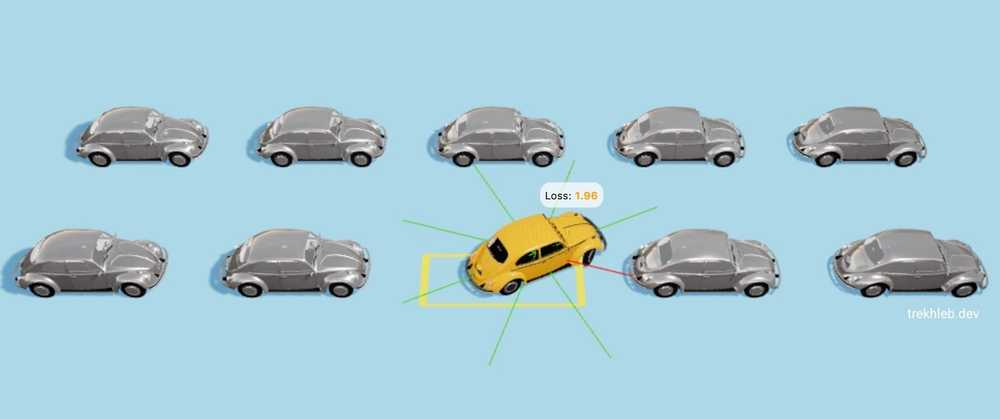## TL;DR

In this article, we'll train the car to do self-parking using a genetic algorithm.

We'll create the 1st generation of cars with random genomes that will behave something like this: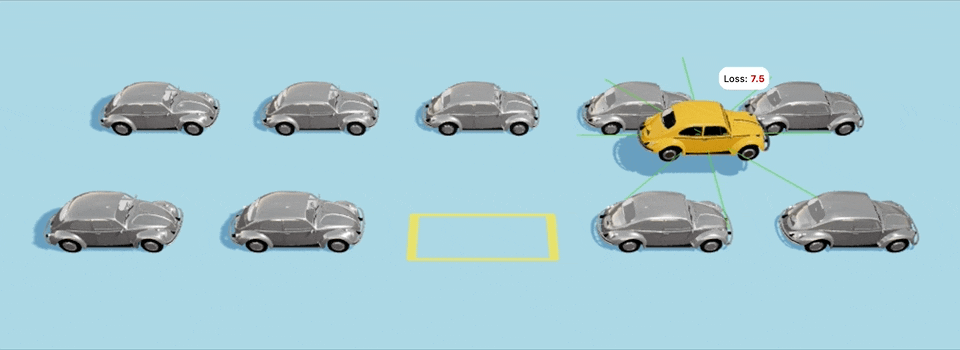On the ≈40th generation the cars start learning what the self-parking is and start getting closer to the parking spot: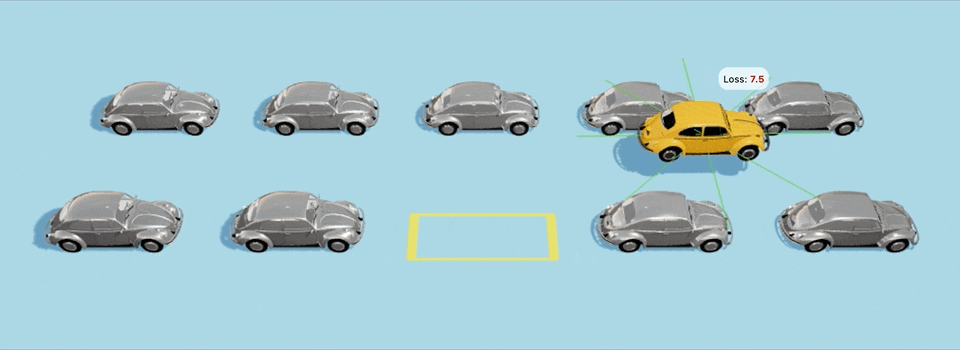Another example with a bit more challenging starting point: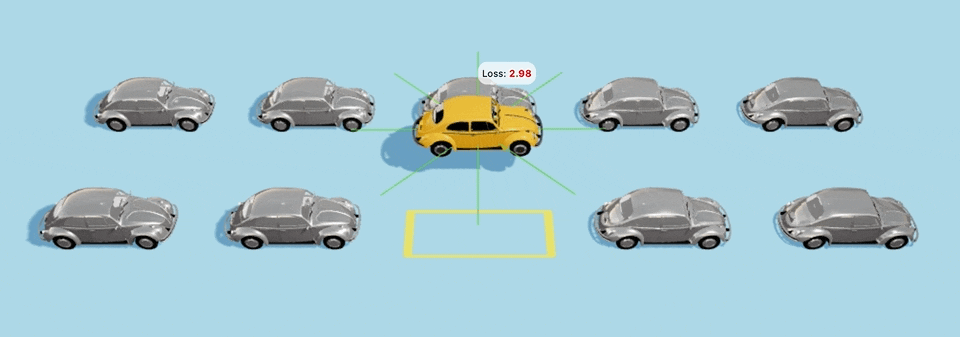Yeah-yeah, the cars are hitting some other cars along the way, and also are not perfectly fitting the parking spot, but this is only the 40th generation since the creation of the world for them, so be merciful and give the cars some space to grow :D

You may launch the 🚕 Self-parking Car Evolution Simulator to see the evolution process directly in your browser. The simulator gives you the following opportunities:

The genetic algorithm for this project is implemented in TypeScript. The full genetic source code will be shown in this article, but you may also find the final code examples in the Evolution Simulator repository.

We're going to use a genetic algorithm for the particular task of evolving cars' genomes. However, this article only touches on the basics of the algorithm and is by no means a complete guide to the genetic algorithm topic.

Having that said, let's deep dive into more details...

## The Plan

Step-by-step we're going to break down a high-level task of creating the self-parking car to the straightforward low-level optimization problem of finding the optimal combination of `180` bits (finding the optimal car genome).

Here is what we're going to do:

1. 💪🏻 Give the muscles (engine, steering wheel) to the car so that it could move towards the parking spot.
2. 👀 Give the eyes (sensors) to the car so that it could see the obstacles around.
3. 🧠 Give the brain to the car that will control the muscles (movements) based on what the car sees (obstacles via sensors). The brain will be simply a pure function `movements = f(sensors)`.
4. 🧬 Evolve the brain to do the right moves based on the sensors input. This is where we will apply a genetic algorithm. Generation after generation our brain function `movements = f(sensors)` will learn how to move the car towards the parking spot.

## Giving the muscles to the car

To be able to move, the car would need "muscles". Let's give the car two types of muscles:

1. Engine muscle - allows the car to move ↓ back, ↑ forth, or ◎ stand steel (neutral gear)
2. Steering wheel muscle - allows the car to turn ← left, → right, or ◎ go straight while moving

With these two muscles the car can perform the following movements: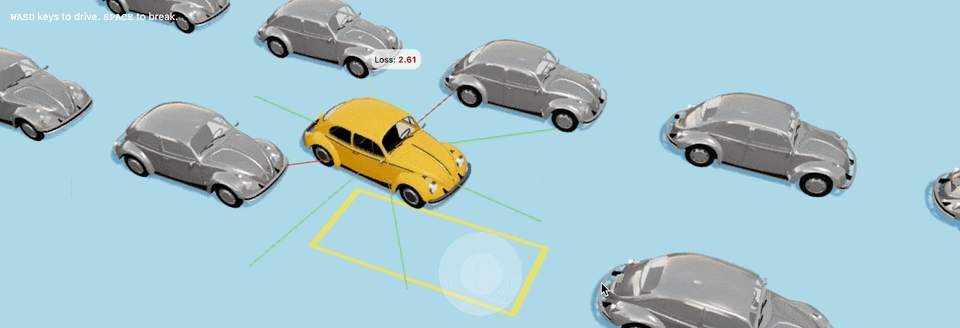In our case, the muscles are receivers of the signals that come from the brain once every `100ms` (milliseconds). Based on the value of the brain's signal the muscles act differently. We'll cover the "brain" part below, but for now, let's say that our brain may send only 3 possible signals to each muscle: `-1`, `0`, or `+1`.

``type MuscleSignal = -1 | 0 | 1;``

For example, the brain may send the signal with the value of `+1` to the engine muscle and it will start moving the car forward. The signal `-1` to the engine moves the car backward. At the same time, if the brain will send the signal of `-1` to the steering wheel muscle, it will turn the car to the left, etc.

Here is how the brain signal values map to the muscle actions in our case:

Muscle`Signal = -1``Signal = 0``Signal = +1`
Engine↓ Backward◎ Neutral↑ Forward
Steering wheel← Left◎ Straight→ Right

You may use the Evolution Simulator and try to park the car manually to see how the car muscles work. Every time you press one of the `WASD` keyboard keys (or use a touch-screen joystick) you send these `-1`, `0`, or `+1` signals to the engine and steering wheel muscles.

## Giving the eyes to the car

Before our car will learn how to do self-parking using its muscles, it needs to be able to "see" the surroundings. Let's give it the `8` eyes in a form of distance sensors:

• Each sensor can detect the obstacle in a distance range of `0-4m` (meters).
• Each sensor reports the latest information about the obstacles it "sees" to the car's "brain" every `100ms`.
• Whenever the sensor doesn't see any obstacles it reports the value of `0`. On the contrary, if the value of the sensor is small but not zero (i.e. `0.01m`) it would mean that the obstacle is close.You may use the Evolution Simulator and see how the color of each sensor changes based on how close the obstacle is.

## Giving the brain to the car

At this moment, our car can "see" and "move", but there is no "coordinator", that would transform the signals from the "eyes" to the proper movements of the "muscles". We need to give the car a "brain".

### Brain input

As an input from the sensors, every `100ms` the brain will be getting `8` float numbers, each one in range of `[0...4]`. For example, the input might look like this:

``````const sensors: Sensors = [s0, s1, s2, s3, s4, s5, s6, s7];
// i.e. 🧠 ← [0, 0.5, 4, 0.002, 0, 3.76, 0, 1.245]``````

### Brain output

Every `100ms` the brain should produce two integers as an output:

1. One number as a signal for the engine: `engineSignal`
2. One number as a signal for the steering wheel: `wheelSignal`

Each number should be of the type `MuscleSignal` and might take one of three values: `-1`, `0`, or `+1`.

### Brain formulas/functions

Keeping in mind the brain's input and output mentioned above we may say that the brain is just a function:

``````const { engineSignal, wheelSignal } = brainToMuscleSignal( brainFunction(sensors)
);
// i.e. { engineSignal: 0, wheelSignal: -1 } ← 🧠 ← [0, 0.5, 4, 0.002, 0, 3.76, 0, 1.245]``````

Where `brainToMuscleSignal()` is a function that converts raw brain signals (any float number) to muscle signals (to `-1`, `0`, or `+1` number) so that muscles could understand it. We'll implement this converter function below.

The main question now is what kind of a function the `brainFunction()` is.

To make the car smarter and its movements to be more sophisticated we could go with a Multilayer Perceptron. The name is a bit scary but this is a simple Neural Network with a basic architecture (think of it as a big formula with many parameters/coefficients).

I've covered Multilayer Perceptrons with a bit more details in my homemade-machine-learning, machine-learning-experiments, and nano-neuron projects. You may even challenge that simple network to recognize your written digits.

However, to avoid the introduction of a whole new concept of Neural Networks, we'll go with a much simpler approach and we'll use two Linear Polynomials with multiple variables (to be more precise, each polynomial will have exactly `8` variables, since we have `8` sensors) which will look something like this:

``````engineSignal = brainToMuscleSignal( (e0 * s0) + (e1 * s1) + ... + (e7 * s7) + e8 // <- brainFunction
) wheelSignal = brainToMuscleSignal( (w0 * s0) + (w1 * s1) + ... + (w7 * s7) + w8 // <- brainFunction
)``````

Where:

• `[s0, s1, ..., s7]` - the `8` variables, which are the `8` sensor values. These are dynamic.
• `[e0, e1, ..., e8]` - the `9` coefficients for the engine polynomial. These the car will need to learn, and they will be static.
• `[w0, w1, ..., w8]` - the `9` coefficients for the steering wheel polynomial. These the car will need to learn, and they will be static

The cost of using the simpler function for the brain will be that the car won't be able to learn some sophisticated moves and also won't be able to generalize well and adapt well to unknown surroundings. But for our particular parking lot and for the sake of demonstrating the work of a genetic algorithm it should still be enough.

We may implement the generic polynomial function in the following way:

``````type Coefficients = number[]; // Calculates the value of a linear polynomial based on the coefficients and variables.
const linearPolynomial = (coefficients: Coefficients, variables: number[]): number => { if (coefficients.length !== (variables.length + 1)) { throw new Error('Incompatible number of polynomial coefficients and variables'); } let result = 0; coefficients.forEach((coefficient: number, coefficientIndex: number) => { if (coefficientIndex < variables.length) { result += coefficient * variables[coefficientIndex]; } else { // The last coefficient needs to be added up without multiplication. result += coefficient } }); return result;
};``````

The car's brain in this case will consist of two polynomials and will look like this:

``````const engineSignal: MuscleSignal = brainToMuscleSignal( linearPolynomial(engineCoefficients, sensors)
); const wheelSignal: MuscleSignal = brainToMuscleSignal( linearPolynomial(wheelCoefficients, sensors)
);``````

The output of a `linearPolynomial()` function is a float number. The `brainToMuscleSignal()` function need to convert the wide range of floats to three particular integers, and it will do it in two steps:

1. Convert the float of a wide range (i.e. `0.456` or `3673.45` or `-280`) to the float in a range of `(0...1)` (i.e. `0.05` or `0.86`)
2. Convert the float in a range of `(0...1)` to one of three integer values of `-1`, `0`, or `+1`. For example, the floats that are close to `0` will be converted to `-1`, the floats that are close to `0.5` will be converted to `0`, and the floats that are close to `1` will be converted to `1`.

To do the first part of the conversion we need to introduce a Sigmoid Function which implements the following formula:It converts the wide range of floats (the `x` axis) to float numbers with a limited range of `(0...1)` (the `y` axis). This is exactly what we need.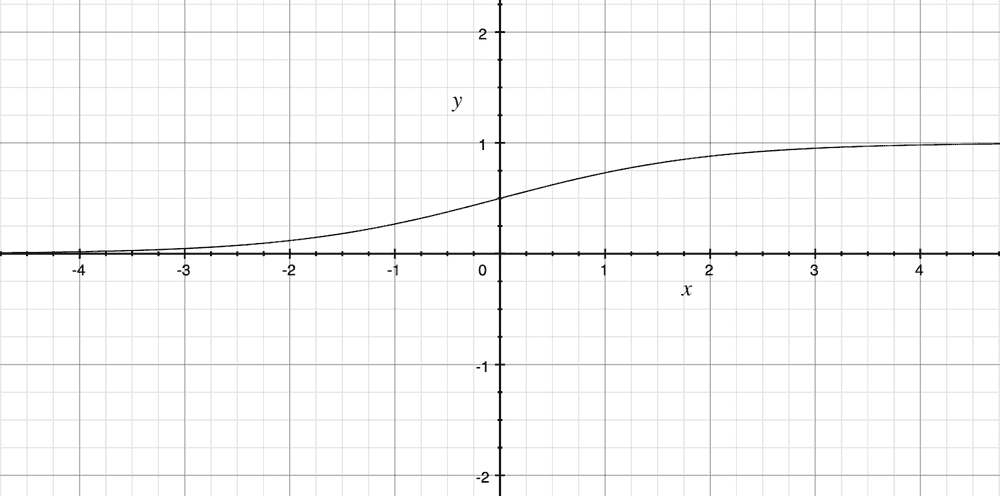Here is how the conversion steps would look on the Sigmoid graph.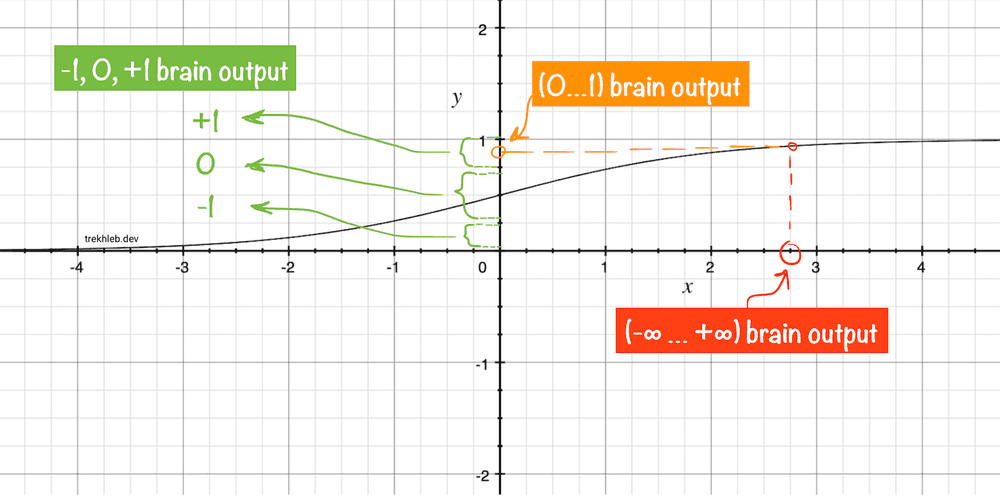The implementation of two conversion steps mentioned above would look like this:

``````// Calculates the sigmoid value for a given number.
const sigmoid = (x: number): number => { return 1 / (1 + Math.E ** -x);
}; // Converts sigmoid value (0...1) to the muscle signals (-1, 0, +1)
// The margin parameter is a value between 0 and 0.5:
// [0 ... (0.5 - margin) ... 0.5 ... (0.5 + margin) ... 1]
const sigmoidToMuscleSignal = (sigmoidValue: number, margin: number = 0.4): MuscleSignal => { if (sigmoidValue < (0.5 - margin)) { return -1; } if (sigmoidValue > (0.5 + margin)) { return 1; } return 0;
}; // Converts raw brain signal to the muscle signal.
const brainToMuscleSignal = (rawBrainSignal: number): MuscleSignal => { const normalizedBrainSignal = sigmoid(rawBrainSignal); return sigmoidToMuscleSignal(normalizedBrainSignal);
}``````

## Car's genome (DNA)

☝🏻 The main conclusion from the "Eyes", "Muscles" and "Brain" sections above should be this: the coefficients `[e0, e1, ..., e8]` and `[w0, w1, ..., w8]` defines the behavior of the car. These `18` numbers together form the unique car's Genome (or car's DNA).

### Car genome in a decimal form

Let's join the `[e0, e1, ..., e8]` and `[w0, w1, ..., w8]` brain coefficients together to form a car's genome in a decimal form:

``````// Car genome as a list of decimal numbers (coefficients).
const carGenomeBase10 = [e0, e1, ..., e8, w0, w1, ..., w8]; // i.e. carGenomeBase10 = [17.5, 0.059, -46, 25, 156, -0.085, -0.207, -0.546, 0.071, -58, 41, 0.011, 252, -3.5, -0.017, 1.532, -360, 0.157]``````

### Car genome in a binary form

Let's move one step deeper (to the level of the genes) and convert the decimal numbers of the car's genome to the binary format (to the plain `1`s and `0`s).

I've described in the detail the process of converting the floating-point numbers to binary numbers in the Binary representation of the floating-point numbers article. You might want to check it out if the code in this section is not clear.

Here is a quick example of how the floating-point number may be converted to the `16 bits` binary number (again, feel free to read this first if the example is confusing):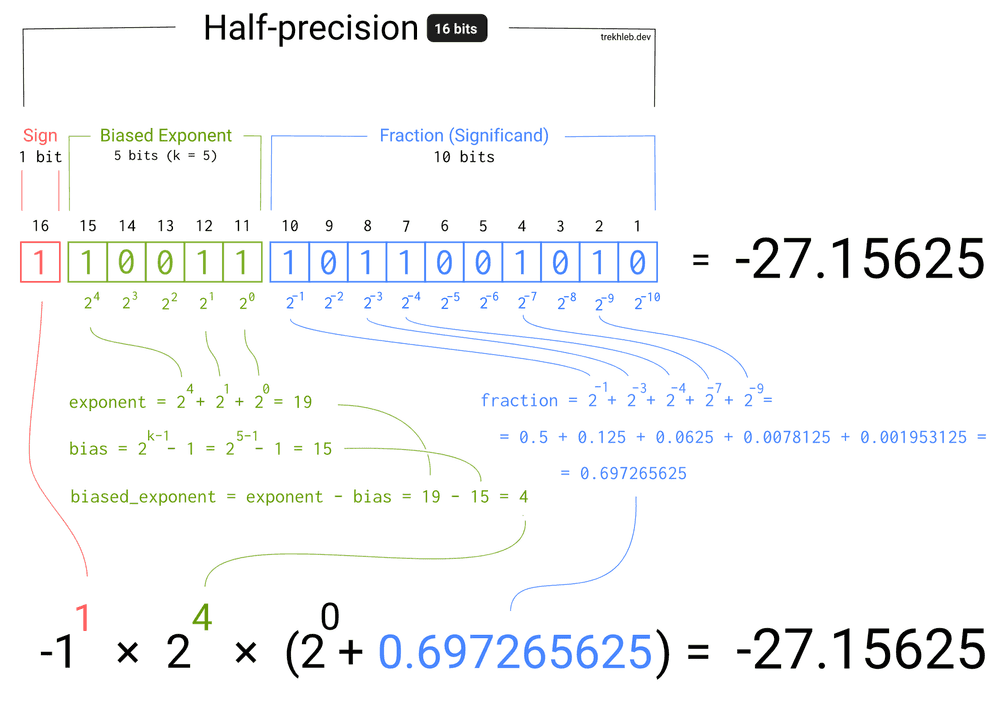In our case, to reduce the genome length, we will convert each floating coefficient to the non-standard `10 bits` binary number (`1` sign bit, `4` exponent bits, `5` fraction bits).

We have `18` coefficients in total, every coefficient will be converted to `10` bits number. It means that the car's genome will be an array of `0`s and `1`s with a length of `18 * 10 = 180 bits`.

For example, for the genome in a decimal format that was mentioned above, its binary representation would look like this:

``````type Gene = 0 | 1; type Genome = Gene[]; const genome: Genome = [ // Engine coefficients. 0, 1, 0, 1, 1, 0, 0, 0, 1, 1, // <- 17.5 0, 0, 0, 1, 0, 1, 1, 1, 0, 0, // <- 0.059 1, 1, 1, 0, 0, 0, 1, 1, 1, 0, // <- -46 0, 1, 0, 1, 1, 1, 0, 0, 1, 0, // <- 25 0, 1, 1, 1, 0, 0, 0, 1, 1, 1, // <- 156 1, 0, 0, 1, 1, 0, 1, 1, 0, 0, // <- -0.085 1, 0, 1, 0, 0, 1, 0, 1, 0, 1, // <- -0.207 1, 0, 1, 1, 0, 0, 0, 0, 1, 1, // <- -0.546 0, 0, 0, 1, 1, 0, 0, 1, 0, 0, // <- 0.071 // Wheels coefficients. 1, 1, 1, 0, 0, 1, 1, 0, 1, 0, // <- -58 0, 1, 1, 0, 0, 0, 1, 0, 0, 1, // <- 41 0, 0, 0, 0, 0, 0, 1, 0, 1, 0, // <- 0.011 0, 1, 1, 1, 0, 1, 1, 1, 1, 1, // <- 252 1, 1, 0, 0, 0, 1, 1, 0, 0, 0, // <- -3.5 1, 0, 0, 0, 1, 0, 0, 1, 0, 0, // <- -0.017 0, 0, 1, 1, 1, 1, 0, 0, 0, 1, // <- 1.532 1, 1, 1, 1, 1, 0, 1, 1, 0, 1, // <- -360 0, 0, 1, 0, 0, 0, 1, 0, 0, 0, // <- 0.157
];``````

Oh my! The binary genome looks so cryptic. But can you imagine, that these `180` zeroes and ones alone define how the car behaves in the parking lot! It's like you hacked someone's DNA and know what each gene means exactly. Amazing!

By the way, you may see the exact values of genomes and coefficients for the best performing car on the Evolution Simulator dashboard: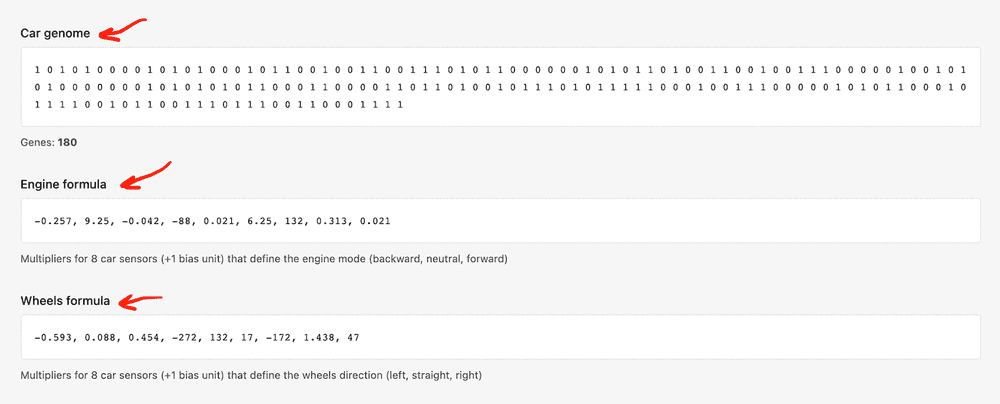Here is the source code that performs the conversion from binary to decimal format for the floating-point numbers (the brain will need it to decode the genome and to produce the muscle signals based on the genome data):

``````type Bit = 0 | 1; type Bits = Bit[]; type PrecisionConfig = { signBitsCount: number, exponentBitsCount: number, fractionBitsCount: number, totalBitsCount: number,
}; type PrecisionConfigs = { custom: PrecisionConfig,
}; const precisionConfigs: PrecisionConfigs = { // Custom-made 10-bits precision for faster evolution progress. custom: { signBitsCount: 1, exponentBitsCount: 4, fractionBitsCount: 5, totalBitsCount: 10, },
}; // Converts the binary representation of the floating-point number to decimal float number.
function bitsToFloat(bits: Bits, precisionConfig: PrecisionConfig): number { const { signBitsCount, exponentBitsCount } = precisionConfig; // Figuring out the sign. const sign = (-1) ** bits; // -1^1 = -1, -1^0 = 1 // Calculating the exponent value. const exponentBias = 2 ** (exponentBitsCount - 1) - 1; const exponentBits = bits.slice(signBitsCount, signBitsCount + exponentBitsCount); const exponentUnbiased = exponentBits.reduce( (exponentSoFar: number, currentBit: Bit, bitIndex: number) => { const bitPowerOfTwo = 2 ** (exponentBitsCount - bitIndex - 1); return exponentSoFar + currentBit * bitPowerOfTwo; }, 0, ); const exponent = exponentUnbiased - exponentBias; // Calculating the fraction value. const fractionBits = bits.slice(signBitsCount + exponentBitsCount); const fraction = fractionBits.reduce( (fractionSoFar: number, currentBit: Bit, bitIndex: number) => { const bitPowerOfTwo = 2 ** -(bitIndex + 1); return fractionSoFar + currentBit * bitPowerOfTwo; }, 0, ); // Putting all parts together to calculate the final number. return sign * (2 ** exponent) * (1 + fraction);
} // Converts the 8-bit binary representation of the floating-point number to decimal float number.
function bitsToFloat10(bits: Bits): number { return bitsToFloat(bits, precisionConfigs.custom);
}``````

### Brain function working with binary genome

Previously our brain function was working with the decimal form of `engineCoefficients` and `wheelCoefficients` polynomial coefficients directly. However, these coefficients are now encoded in the binary form of a genome. Let's add a `decodeGenome()` function that will extract coefficients from the genome and let's rewrite our brain functions:

``````// Car has 16 distance sensors.
const CAR_SENSORS_NUM = 8; // Additional formula coefficient that is not connected to a sensor.
const BIAS_UNITS = 1; // How many genes do we need to encode each numeric parameter for the formulas.
const GENES_PER_NUMBER = precisionConfigs.custom.totalBitsCount; // Based on 8 distance sensors we need to provide two formulas that would define car's behavior:
// 1. Engine formula (input: 8 sensors; output: -1 (backward), 0 (neutral), +1 (forward))
// 2. Wheels formula (input: 8 sensors; output: -1 (left), 0 (straight), +1 (right))
const ENGINE_FORMULA_GENES_NUM = (CAR_SENSORS_NUM + BIAS_UNITS) * GENES_PER_NUMBER;
const WHEELS_FORMULA_GENES_NUM = (CAR_SENSORS_NUM + BIAS_UNITS) * GENES_PER_NUMBER; // The length of the binary genome of the car.
const GENOME_LENGTH = ENGINE_FORMULA_GENES_NUM + WHEELS_FORMULA_GENES_NUM; type DecodedGenome = { engineFormulaCoefficients: Coefficients, wheelsFormulaCoefficients: Coefficients,
} // Converts the genome from a binary form to the decimal form.
const genomeToNumbers = (genome: Genome, genesPerNumber: number): number[] => { if (genome.length % genesPerNumber !== 0) { throw new Error('Wrong number of genes in the numbers genome'); } const numbers: number[] = []; for (let numberIndex = 0; numberIndex < genome.length; numberIndex += genesPerNumber) { const number: number = bitsToFloat10(genome.slice(numberIndex, numberIndex + genesPerNumber)); numbers.push(number); } return numbers;
}; // Converts the genome from a binary form to the decimal form
// and splits the genome into two sets of coefficients (one set for each muscle).
const decodeGenome = (genome: Genome): DecodedGenome => { const engineGenes: Gene[] = genome.slice(0, ENGINE_FORMULA_GENES_NUM); const wheelsGenes: Gene[] = genome.slice( ENGINE_FORMULA_GENES_NUM, ENGINE_FORMULA_GENES_NUM + WHEELS_FORMULA_GENES_NUM, ); const engineFormulaCoefficients: Coefficients = genomeToNumbers(engineGenes, GENES_PER_NUMBER); const wheelsFormulaCoefficients: Coefficients = genomeToNumbers(wheelsGenes, GENES_PER_NUMBER); return { engineFormulaCoefficients, wheelsFormulaCoefficients, };
}; // Update brain function for the engine muscle.
export const getEngineMuscleSignal = (genome: Genome, sensors: Sensors): MuscleSignal => { const {engineFormulaCoefficients: coefficients} = decodeGenome(genome); const rawBrainSignal = linearPolynomial(coefficients, sensors); return brainToMuscleSignal(rawBrainSignal);
}; // Update brain function for the wheels muscle.
export const getWheelsMuscleSignal = (genome: Genome, sensors: Sensors): MuscleSignal => { const {wheelsFormulaCoefficients: coefficients} = decodeGenome(genome); const rawBrainSignal = linearPolynomial(coefficients, sensors); return brainToMuscleSignal(rawBrainSignal);
};``````

## Self-driving car problem statement

☝🏻 So, finally, we've got to the point when the high-level problem of making the car to be a self-parking car is broken down to the straightforward optimization problem of finding the optimal combination of `180` ones and zeros (finding the "good enough" car's genome). Sounds simple, doesn't it?

### Naive approach

We could approach the problem of finding the "good enough" genome in a naive way and try out all possible combinations of genes:

1. `[0, ..., 0, 0]`, and then...
2. `[0, ..., 0, 1]`, and then...
3. `[0, ..., 1, 0]`, and then...
4. `[0, ..., 1, 1]`, and then...
5. ...

But, let's do some math. With `180` bits and with each bit being equal either to `0` or to `1` we would have `2^180` (or `1.53 * 10^54`) possible combinations. Let's say we would need to give `15s` to each car to see if it will park successfully or not. Let's also say that we may run a simulation for `10` cars at once. Then we would need `15 * (1.53 * 10^54) / 10 = 2.29 * 10^54 [seconds]` which is `7.36 * 10^46 [years]`. Pretty long waiting time. Just as a side thought, it is only `2.021 * 10^3 [years]` that have passed after Christ was born.

### Genetic approach

We need a faster algorithm to find the optimal value of the genome. This is where the genetic algorithm comes to the rescue. We might not find the best value of the genome, but there is a chance that we may find the optimal value of it. And, what is, more importantly, we don't need to wait that long. With the Evolution Simulator I was able to find a pretty good genome within `24 [hours]`.

## Genetic algorithm basics

A genetic algorithms (GA) inspired by the process of natural selection, and are commonly used to generate high-quality solutions to optimization problems by relying on biologically inspired operators such as crossover, mutation and selection.

The problem of finding the "good enough" combination of genes for the car looks like an optimization problem, so there is a good chance that GA will help us here.

We're not going to cover a genetic algorithm in all details, but on a high level here are the basic steps that we will need to do:

1. CREATE – the very first generation of cars can't come out of nothing, so we will generate a set of random car genomes (set of binary arrays with the length of `180`) at the very beginning. For example, we may create `~1000` cars. With a bigger population the chances to find the optimal solution (and to find it faster) increase.
2. SELECT - we will need to select the fittest individuums out of the current generation for further mating (see the next step). The fitness of each individuum will be defined based on the fitness function, which in our case, will show how close the car approached the target parking spot. The closer the car to the parking spot, the fitter it is.
3. MATE – simply saying we will allow the selected "♂ father-cars" to have "sex" with the selected "♀ mother-cars" so that their genomes could mix in a `~50/50` proportion and produce "♂♀ children-cars" genomes. The idea is that the children cars might get better (or worse) in self-parking, by taking the best (or the worst) bits from their parents.
4. MUTATE - during the mating process some genes may randomly mutate (`1`s and `0`s in child genome may flip). This may bring a wider variety of children genomes and, thus, a wider variety of children cars behavior. Imagine that the 1st bit was accidentally set to `0` for all `~1000` cars. The only way to try the car with the 1st bit being set to `1` is through the random mutations. At the same time, extensive mutations may ruin healthy genomes.
5. Go to "Step 2" unless the number of generations has reached the limit (i.e. `100` generations have passed) or unless the top-performing individuums have reached the expected fitness function value (i.e. the best car has approached the parking spot closer than `1 meter`). Otherwise, quit.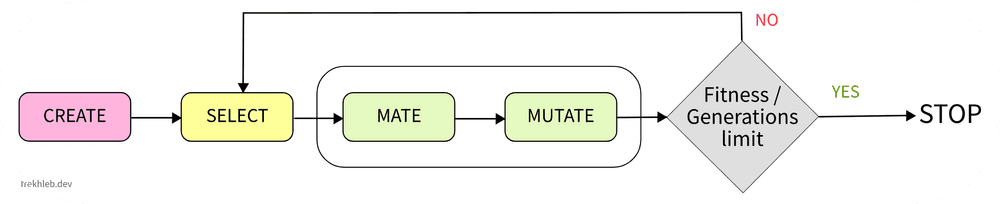## Evolving the car's brain using a Genetic Algorithm

Before launching the genetic algorithm let's go and create the functions for the "CREATE", "SELECT", "MATE" and "MUTATE" steps of the algorithm.

### Functions for the CREATE step

The `createGeneration()` function will create an array of random genomes (a.k.a. population or generation) and will accept two parameters:

• `generationSize` - defines the size of the generation. This generation size will be preserved from generation to generation.
• `genomeLength` - defines the genome length of each individuum in the cars population. In our case, the length of the genome will be `180`.

There is a `50/50` chance for each gene of a genome to be either `0` or `1`.

``````type Generation = Genome[]; type GenerationParams = { generationSize: number, genomeLength: number,
}; function createGenome(length: number): Genome { return new Array(length) .fill(null) .map(() => (Math.random() < 0.5 ? 0 : 1));
} function createGeneration(params: GenerationParams): Generation { const { generationSize, genomeLength } = params; return new Array(generationSize) .fill(null) .map(() => createGenome(genomeLength));
}``````

### Functions for the MUTATE step

The `mutate()` function will mutate some genes randomly based on the `mutationProbability` value.

For example, if the `mutationProbability = 0.1` then there is a `10%` chance for each genome to be mutated. Let's say if we would have a genome of length `10` that looks like `[0, 0, 0, 0, 0, 0 ,0 ,0 ,0 ,0]`, then after the mutation, there will be a chance that 1 gene will be mutated and we may get a genome that might look like `[0, 0, 0, 1, 0, 0 ,0 ,0 ,0 ,0]`.

``````// The number between 0 and 1.
type Probability = number; // @see: https://en.wikipedia.org/wiki/Mutation_(genetic_algorithm)
function mutate(genome: Genome, mutationProbability: Probability): Genome { for (let geneIndex = 0; geneIndex < genome.length; geneIndex += 1) { const gene: Gene = genome[geneIndex]; const mutatedGene: Gene = gene === 0 ? 1 : 0; genome[geneIndex] = Math.random() < mutationProbability ? mutatedGene : gene; } return genome;
}``````

### Functions for the MATE step

The `mate()` function will accept the `father` and the `mother` genomes and will produce two children. We will imitate the real-world scenario and also do the mutation during the mating.

Each bit of the child genome will be defined based on the values of the correspondent bit of the father's or mother's genomes. There is a `50/50%` probability that the child will inherit the bit of the father or the mother. For example, let's say we have genomes of length `4` (for simplicity reasons):

``````Father's genome: [0, 0, 1, 1]
Mother's genome: [0, 1, 0, 1] ↓ ↓ ↓ ↓
Possible kid #1: [0, 1, 1, 1]
Possible kid #2: [0, 0, 1, 1]``````

In the example above the mutation were not taken into account.

Here is the function implementation:

``````// Performs Uniform Crossover: each bit is chosen from either parent with equal probability.
// @see: https://en.wikipedia.org/wiki/Crossover_(genetic_algorithm)
function mate( father: Genome, mother: Genome, mutationProbability: Probability,
): [Genome, Genome] { if (father.length !== mother.length) { throw new Error('Cannot mate different species'); } const firstChild: Genome = []; const secondChild: Genome = []; // Conceive children. for (let geneIndex = 0; geneIndex < father.length; geneIndex += 1) { firstChild.push( Math.random() < 0.5 ? father[geneIndex] : mother[geneIndex] ); secondChild.push( Math.random() < 0.5 ? father[geneIndex] : mother[geneIndex] ); } return [ mutate(firstChild, mutationProbability), mutate(secondChild, mutationProbability), ];
}``````

### Functions for the SELECT step

To select the fittest individuums for further mating we need a way to find out the fitness of each genome. To do this we will use a so-called fitness function.

The fitness function is always related to the particular task that we try to solve, and it is not generic. In our case, the fitness function will measure the distance between the car and the parking spot. The closer the car to the parking spot, the fitter it is. We will implement the fitness function a bit later, but for now, let's introduce the interface for it:

``type FitnessFunction = (genome: Genome) => number;``

Now, let's say we have fitness values for each individuum in the population. Let's also say that we sorted all individuums by their fitness values so that the first individuums are the strongest ones. How should we select the fathers and the mothers from this array? We need to do the selection in a way, that the higher the fitness value of the individuum, the higher the chances of this individuum being selected for mating. The `weightedRandom()` function will help us with this.

``````// Picks the random item based on its weight.
// The items with a higher weight will be picked more often.
const weightedRandom = <T>(items: T[], weights: number[]): { item: T, index: number } => { if (items.length !== weights.length) { throw new Error('Items and weights must be of the same size'); } // Preparing the cumulative weights array. // For example: // - weights = [1, 4, 3] // - cumulativeWeights = [1, 5, 8] const cumulativeWeights: number[] = []; for (let i = 0; i < weights.length; i += 1) { cumulativeWeights[i] = weights[i] + (cumulativeWeights[i - 1] || 0); } // Getting the random number in a range [0...sum(weights)] // For example: // - weights = [1, 4, 3] // - maxCumulativeWeight = 8 // - range for the random number is [0...8] const maxCumulativeWeight = cumulativeWeights[cumulativeWeights.length - 1]; const randomNumber = maxCumulativeWeight * Math.random(); // Picking the random item based on its weight. // The items with higher weight will be picked more often. for (let i = 0; i < items.length; i += 1) { if (cumulativeWeights[i] >= randomNumber) { return { item: items[i], index: i, }; } } return { item: items[items.length - 1], index: items.length - 1, };
};``````

The usage of this function is pretty straightforward. Let's say you really like bananas and want to eat them more often than strawberries. Then you may call `const fruit = weightedRandom(['banana', 'strawberry'], [9, 1])`, and in `≈9` out of `10` cases the `fruit` variable will be equal to `banana`, and only in `≈1` out of `10` times it will be equal to `strawberry`.

To avoid losing the best individuums (let's call them champions) during the mating process we may also introduce a so-called `longLivingChampionsPercentage` parameter. For example, if the `longLivingChampionsPercentage = 10`, then `10%` of the best cars from the previous population will be carried over to the new generation. You may think about it as there are some long-living individuums that can live a long life and see their children and even grandchildren.

Here is the actual implementation of the `select()` function:

``````// The number between 0 and 100.
type Percentage = number; type SelectionOptions = { mutationProbability: Probability, longLivingChampionsPercentage: Percentage,
}; // @see: https://en.wikipedia.org/wiki/Selection_(genetic_algorithm)
function select( generation: Generation, fitness: FitnessFunction, options: SelectionOptions,
) { const { mutationProbability, longLivingChampionsPercentage, } = options; const newGeneration: Generation = []; const oldGeneration = [...generation]; // First one - the fittest one. oldGeneration.sort((genomeA: Genome, genomeB: Genome): number => { const fitnessA = fitness(genomeA); const fitnessB = fitness(genomeB); if (fitnessA < fitnessB) { return 1; } if (fitnessA > fitnessB) { return -1; } return 0; }); // Let long-liver champions continue living in the new generation. const longLiversCount = Math.floor(longLivingChampionsPercentage * oldGeneration.length / 100); if (longLiversCount) { oldGeneration.slice(0, longLiversCount).forEach((longLivingGenome: Genome) => { newGeneration.push(longLivingGenome); }); } // Get the data about he fitness of each individuum. const fitnessPerOldGenome: number[] = oldGeneration.map((genome: Genome) => fitness(genome)); // Populate the next generation until it becomes the same size as a old generation. while (newGeneration.length < generation.length) { // Select random father and mother from the population. // The fittest individuums have higher chances to be selected. let father: Genome | null = null; let fatherGenomeIndex: number | null = null; let mother: Genome | null = null; let matherGenomeIndex: number | null = null; // To produce children the father and mother need each other. // It must be two different individuums. while (!father || !mother || fatherGenomeIndex === matherGenomeIndex) { const { item: randomFather, index: randomFatherGenomeIndex, } = weightedRandom<Genome>(generation, fitnessPerOldGenome); const { item: randomMother, index: randomMotherGenomeIndex, } = weightedRandom<Genome>(generation, fitnessPerOldGenome); father = randomFather; fatherGenomeIndex = randomFatherGenomeIndex; mother = randomMother; matherGenomeIndex = randomMotherGenomeIndex; } // Let father and mother produce two children. const [firstChild, secondChild] = mate(father, mother, mutationProbability); newGeneration.push(firstChild); // Depending on the number of long-living champions it is possible that // there will be the place for only one child, sorry. if (newGeneration.length < generation.length) { newGeneration.push(secondChild); } } return newGeneration;
}``````

### Fitness function

The fitness of the car will be defined by the distance from the car to the parking spot. The higher the distance, the lower the fitness.

The final distance we will calculate is an average distance from `4` car wheels to the correspondent `4` corners of the parking spot. This distance we will call the `loss` which is inversely proportional to the `fitness`.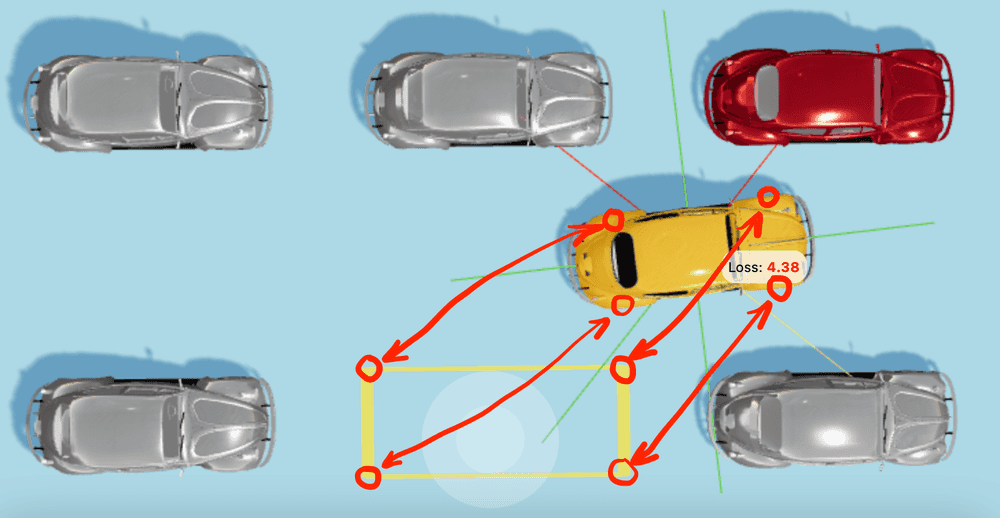Calculating the distance between each wheel and each corner separately (instead of just calculating the distance from the car center to the parking spot center) will make the car preserve the proper orientation relative to the parking spot.

The distance between two points in space will be calculated based on the Pythagorean theorem like this:

``````type NumVec3 = [number, number, number]; // Calculates the XZ distance between two points in space.
// The vertical Y distance is not being taken into account.
const euclideanDistance = (from: NumVec3, to: NumVec3) => { const fromX = from; const fromZ = from; const toX = to; const toZ = to; return Math.sqrt((fromX - toX) ** 2 + (fromZ - toZ) ** 2);
};``````

The distance (the `loss`) between the car and the parking spot will be calculated like this:

``````type RectanglePoints = { fl: NumVec3, // Front-left fr: NumVec3, // Front-right bl: NumVec3, // Back-left br: NumVec3, // Back-right
}; type GeometricParams = { wheelsPosition: RectanglePoints, parkingLotCorners: RectanglePoints,
}; const carLoss = (params: GeometricParams): number => { const { wheelsPosition, parkingLotCorners } = params; const { fl: flWheel, fr: frWheel, br: brWheel, bl: blWheel, } = wheelsPosition; const { fl: flCorner, fr: frCorner, br: brCorner, bl: blCorner, } = parkingLotCorners; const flDistance = euclideanDistance(flWheel, flCorner); const frDistance = euclideanDistance(frWheel, frCorner); const brDistance = euclideanDistance(brWheel, brCorner); const blDistance = euclideanDistance(blWheel, blCorner); return (flDistance + frDistance + brDistance + blDistance) / 4;
};``````

Since the `fitness` should be inversely proportional to the `loss` we'll calculate it like this:

``````const carFitness = (params: GeometricParams): number => { const loss = carLoss(params); // Adding +1 to avoid a division by zero. return 1 / (loss + 1);
};``````

You may see the `fitness` and the `loss` values for a specific genome and for a current car position on the Evolution Simulator dashboard: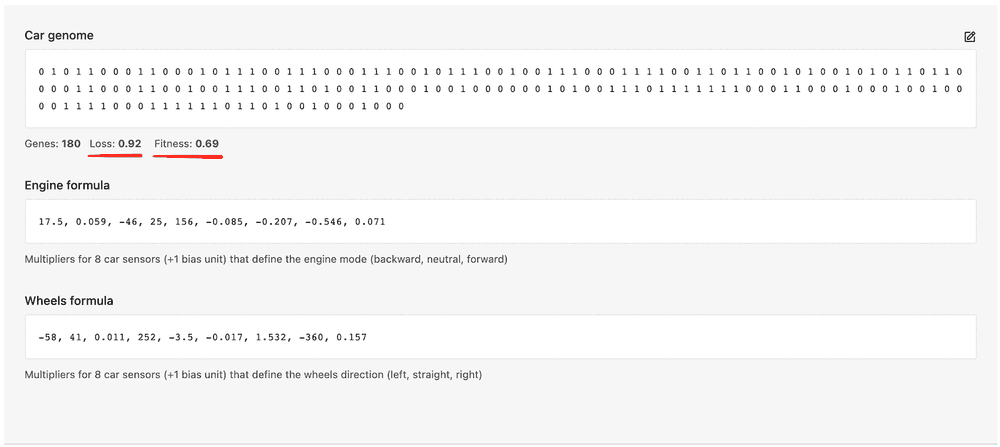## Launching the evolution

Let's put the evolution functions together. We're going to "create the world", launch the evolution loop, make the time going, the generation evolving, and the cars learning how to park.

To get the fitness values of each car we need to run a simulation of the cars behavior in a virtual 3D world. The Evolution Simulator does exactly that - it runs the code below in the simulator, which is made with Three.js:

``````// Evolution setup example.
// Configurable via the Evolution Simulator.
const GENERATION_SIZE = 1000;
const LONG_LIVING_CHAMPIONS_PERCENTAGE = 6;
const MUTATION_PROBABILITY = 0.04;
const MAX_GENERATIONS_NUM = 40; // Fitness function.
// It is like an annual doctor's checkup for the cars.
const carFitnessFunction = (genome: Genome): number => { // The evolution simulator calculates and stores the fitness values for each car in the fitnessValues map. // Here we will just fetch the pre-calculated fitness value for the car in current generation. const genomeKey = genome.join(''); return fitnessValues[genomeKey];
}; // Creating the "world" with the very first cars generation.
let generationIndex = 0;
let generation: Generation = createGeneration({ generationSize: GENERATION_SIZE, genomeLength: GENOME_LENGTH, // <- 180 genes
}); // Starting the "time".
while(generationIndex < MAX_GENERATIONS_NUM) { // SIMULATION IS NEEDED HERE to pre-calculate the fitness values. // Selecting, mating, and mutating the current generation. generation = select( generation, carFitnessFunction, { mutationProbability: MUTATION_PROBABILITY, longLivingChampionsPercentage: LONG_LIVING_CHAMPIONS_PERCENTAGE, }, ); // Make the "time" go by. generationIndex += 1;
} // Here we may check the fittest individuum of the latest generation.
const fittestCar = generation;``````

After running the `select()` function, the `generation` array is sorted by the fitness values in descending order. Therefore, the fittest car will always be the first car in the array.

The 1st generation of cars with random genomes will behave something like this:On the ≈40th generation the cars start learning what the self-parking is and start getting closer to the parking spot:Another example with a bit more challenging starting point:The cars are hitting some other cars along the way, and also are not perfectly fitting the parking spot, but this is only the 40th generation since the creation of the world for them, so you may give the cars some more time to learn.

From generation to generation we may see how the loss values are going down (which means that fitness values are going up). The `P50 Avg Loss` shows the average loss value (average distance from the cars to the parking spot) of the `50%` of fittest cars. The `Min Loss` shows the loss value of the fittest car in each generation.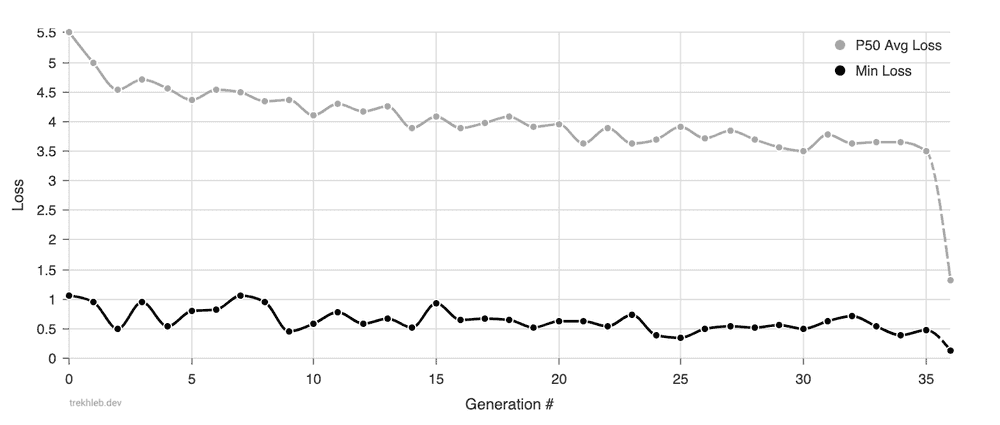You may see that on average the `50%` of the fittest cars of the generation are learning to get closer to the parking spot (from `5.5m` away from the parking spot to `3.5m` in 35 generations). The trend for the `Min Loss` values is less obvious (from `1m` to `0.5m` with some noise signals), however from the animations above you may see that cars have learned some basic parking moves.

## Conclusion

In this article, we've broken down the high-level task of creating the self-parking car to the straightforward low-level task of finding the optimal combination of `180` ones and zeroes (finding the optimal car genome).

Then we've applied the genetic algorithm to find the optimal car genome. It allowed us to get pretty good results in several hours of simulation (instead of many years of running the naive approach).

You may launch the 🚕 Self-parking Car Evolution Simulator to see the evolution process directly in your browser. The simulator gives you the following opportunities:

The full genetic source code that was shown in this article may also be found in the Evolution Simulator repository. If you are one of those folks who will actually count and check the number of lines to make sure there are less than 500 of them (excluding tests), please feel free to check the code here 🥸.

There are still some unresolved issues with the code and the simulator:

• The car's brain is oversimplified and it uses linear equations instead of, let's say, neural networks. It makes the car not adaptable to the new surroundings or to the new parking lot types.
• We don't decrease the car's fitness value when the car is hitting the other car. Therefore the car doesn't "feel" any guilt in creating the road accident.
• The evolution simulator is not stable. It means that the same car genome may produce different fitness values, which makes the evolution less efficient.
• The evolution simulator is also very heavy in terms of performance, which slows down the evolution progress since we can't train, let's say, 1000 cars at once.
• Also the Evolution Simulator requires the browser tab to be open and active to perform the simulation.
• and more...

However, the purpose of this article was to have some fun while learning how the genetic algorithm works and not to build a production-ready self-parking Teslas. So, even with the issues mentioned above, I hope you've had a good time going through the article.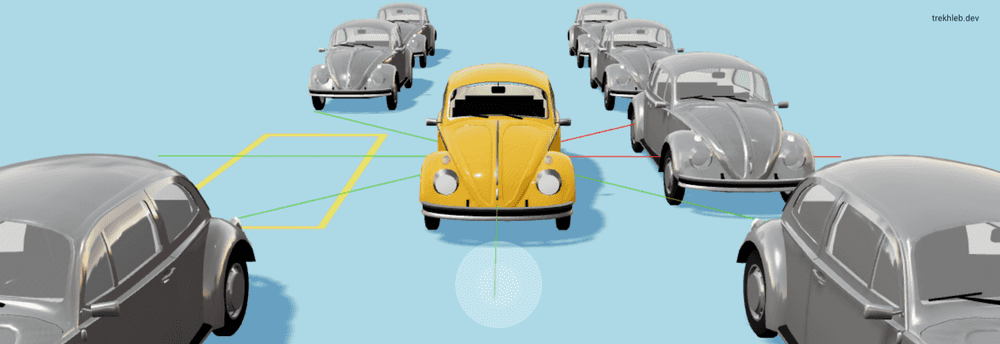Get my latest posts and project updates by email